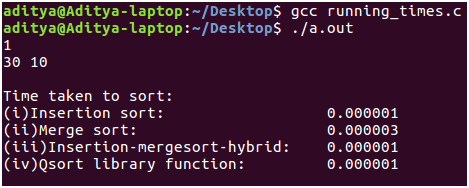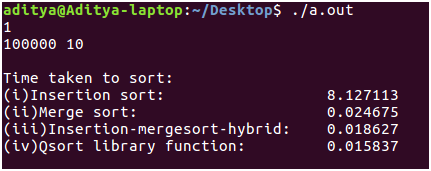# Asymptotic Analysis and comparison of sorting algorithms

• Difficulty Level : Medium
• Last Updated : 11 Jul, 2022

It is a well established fact that merge sort runs faster than insertion sort. Using asymptotic analysis we can prove that merge sort runs in O(nlogn) time and insertion sort takes O(n^2). It is obvious because merge sort uses a divide-and-conquer approach by recursively solving the problems where as insertion sort follows an incremental approach.
If we scrutinize the time complexity analysis even further, we’ll get to know that insertion sort isn’t that bad enough. Surprisingly, insertion sort beats merge sort on smaller input size. This is because there are few constants which we ignore while deducing the time complexity. On larger input sizes of the order 10^4 this doesn’t influence the behavior of our function. But when input sizes fall below, say less than 40, then the constants in the equation dominate the input size ‘n’.
So far, so good. But I wasn’t satisfied with such mathematical analysis. As a computer science undergrad we must believe in writing code. I’ve written a C program to get a feel of how the algorithms compete against each other for various input sizes. And also, why such rigorous mathematical analysis is done on establishing running time complexities of these sorting algorithms.

Implementation:

 `//C++ code to compare performance of sorting algorithms``#include ``#include ``#include ``#include ``#define MAX_ELEMENT_IN_ARRAY 1000000001`` ` `int` `cmpfunc (``const` `void` `* a, ``const` `void` `* b)``{``    ``// Compare function used by qsort``    ``return` `( *(``int``*)a - *(``int``*)b );``}`` ` `int``* generate_random_array(``int` `n)``{``    ``srand``(``time``(NULL));``    ``int` `*a = ``malloc``(``sizeof``(``int``) * n), i;``    ``for``(i = 0; i < n; ++i)``        ``a[i] = ``rand``() % MAX_ELEMENT_IN_ARRAY;``    ``return` `a;``}`` ` `int``* copy_array(``int` `a[], ``int` `n)``{``    ``int` `*arr = ``malloc``(``sizeof``(``int``) * n);``    ``int` `i;``    ``for``(i = 0; i < n ;++i)``        ``arr[i] = a[i];``    ``return` `arr;``}`` ` `//Code for Insertion Sort``void` `insertion_sort_asc(``int` `a[], ``int` `start, ``int` `end)``{``    ``int` `i;``    ``for``(i = start + 1; i <= end ; ++i)``    ``{``        ``int` `key = a[i];``        ``int` `j = i - 1;``        ``while``(j >= start && a[j] > key)``        ``{``            ``a[j + 1] = a[j];``            ``--j;``        ``}``        ``a[j + 1] = key;``    ``}``}`` ` `//Code for Merge Sort``void` `merge(``int` `a[], ``int` `start, ``int` `end, ``int` `mid)``{``    ``int` `i = start, j = mid + 1, k = 0;``    ``int` `*aux = ``malloc``(``sizeof``(``int``) * (end - start + 1));``    ``while``(i <= mid && j <= end)``    ``{``        ``if``(a[i] <= a[j])``            ``aux[k++] = a[i++];``        ``else``            ``aux[k++] = a[j++];``    ``}``    ``while``(i <= mid)``        ``aux[k++] = a[i++];``    ``while``(j <= end)``        ``aux[k++] = a[j++];``    ``j = 0;``    ``for``(i = start;i <= end;++i)``        ``a[i] = aux[j++];``    ``free``(aux);``}`` ` `void` `_merge_sort(``int` `a[],``int` `start,``int` `end)``{``    ``if``(start < end)``    ``{``        ``int` `mid = start + (end - start) / 2;``        ``_merge_sort(a,start,mid);``        ``_merge_sort(a,mid + 1,end);``        ``merge(a,start,end,mid);``    ``}``}``void` `merge_sort(``int` `a[],``int` `n)``{``    ``return` `_merge_sort(a,0,n - 1);``}`` ` ` ` `void` `insertion_and_merge_sort_combine(``int` `a[], ``int` `start, ``int` `end, ``int` `k)``{``    ``// Performs insertion sort if size of array is less than or equal to k``    ``// Otherwise, uses mergesort``    ``if``(start < end)``    ``{``        ``int` `size = end - start + 1;``         ` `        ``if``(size <= k)``        ``{``            ``//printf("Performed insertion sort- start = %d and end = %d\n", start, end);``            ``return` `insertion_sort_asc(a,start,end);``        ``}``        ``int` `mid = start + (end - start) / 2;``        ``insertion_and_merge_sort_combine(a,start,mid,k);``        ``insertion_and_merge_sort_combine(a,mid + 1,end,k);``        ``merge(a,start,end,mid);``    ``}``}`` ` `void` `test_sorting_runtimes(``int` `size,``int` `num_of_times)``{``    ``// Measuring the runtime of the sorting algorithms``    ``int` `number_of_times = num_of_times;``    ``int` `t = number_of_times;``    ``int` `n = size;``    ``double` `insertion_sort_time = 0, merge_sort_time = 0;``    ``double` `merge_sort_and_insertion_sort_mix_time = 0, qsort_time = 0;``    ``while``(t--)``    ``{``        ``clock_t` `start, end;``         ` `        ``int` `*a = generate_random_array(n);``        ``int` `*b = copy_array(a,n);``        ``start = ``clock``();``        ``insertion_sort_asc(b,0,n-1);``        ``end = ``clock``();``        ``insertion_sort_time += ((``double``) (end - start)) / CLOCKS_PER_SEC;``        ``free``(b);``        ``int` `*c = copy_array(a,n);``        ``start = ``clock``();``        ``merge_sort(c,n);``        ``end = ``clock``();``        ``merge_sort_time += ((``double``) (end - start)) / CLOCKS_PER_SEC;``        ``free``(c);``        ``int` `*d = copy_array(a,n);``        ``start = ``clock``();``        ``insertion_and_merge_sort_combine(d,0,n-1,40);``        ``end = ``clock``();``        ``merge_sort_and_insertion_sort_mix_time+=((``double``) (end - start))/CLOCKS_PER_SEC;``        ``free``(d);``        ``start = ``clock``();``        ``qsort``(a,n,``sizeof``(``int``),cmpfunc);``        ``end = ``clock``();``        ``qsort_time += ((``double``) (end - start)) / CLOCKS_PER_SEC;``        ``free``(a);``    ``}``     ` `    ``insertion_sort_time /= number_of_times;``    ``merge_sort_time /= number_of_times;``    ``merge_sort_and_insertion_sort_mix_time /= number_of_times;``    ``qsort_time /= number_of_times;``    ``printf``(``"\nTime taken to sort:\n"``            ``"%-35s %f\n"``            ``"%-35s %f\n"``            ``"%-35s %f\n"``            ``"%-35s %f\n\n"``,``            ``"(i)Insertion sort: "``,``            ``insertion_sort_time,``            ``"(ii)Merge sort: "``,``            ``merge_sort_time,``            ``"(iii)Insertion-mergesort-hybrid: "``,``            ``merge_sort_and_insertion_sort_mix_time,``            ``"(iv)Qsort library function: "``,``            ``qsort_time);``}`` ` `int` `main(``int` `argc, ``char` `const` `*argv[])``{``    ``int` `t;``    ``scanf``(``"%d"``, &t);``    ``while``(t--)``    ``{``        ``int` `size, num_of_times;``        ``scanf``(``"%d %d"``, &size, &num_of_times);``        ``test_sorting_runtimes(size,num_of_times);``    ``}``    ``return` `0;``}`

I have compared the running times of the following algorithms:

• Insertion sort: The traditional algorithm with no modifications/optimisation. It performs very well for smaller input sizes. And yes, it does beat merge sort
• Merge sort: Follows the divide-and-conquer approach. For input sizes of the order 10^5 this algorithm is of the right choice. It renders insertion sort impractical for such large input sizes.
• Combined version of insertion sort and merge sort: I have tweaked the logic of merge sort a little bit to achieve a considerably better running time for smaller input sizes. As we know, merge sort splits its input into two halves until it is trivial enough to sort the elements. But here, when the input size falls below a threshold such as ’n’ < 40 then this hybrid algorithm makes a call to traditional insertion sort procedure. From the fact that insertion sort runs faster on smaller inputs and merge sort runs faster on larger inputs, this algorithm makes best use both the worlds.
• Quick sort: I have not implemented this procedure. This is the library function qsort() which is available in . I have considered this algorithm in order to know the significance of implementation. It requires a great deal of programming expertise to minimize the number of steps and make at most use of the underlying language primitives to implement an algorithm in the best way possible. This is the main reason why it is recommended to use library functions. They are written to handle anything and everything. They optimize to the maximum extent possible. And before I forget, from my analysis qsort() runs blazingly fast on virtually any input size!

The Analysis:

• Input: The user has to supply the number of times he/she wants to test the algorithm corresponding to number of test cases. For each test case the user must enter two space separated integers denoting the input size ’n’ and the ‘num_of_times’ denoting the number of times he/she wants to run the analysis and take average. (Clarification: If ‘num_of_times’ is 10 then each of the algorithm specified above runs 10 times and the average is taken. This is done because the input array is generated randomly corresponding to the input size which you specify. The input array could be all sorted. Our it could correspond to the worst case .i.e. descending order. In order to avoid running times of such input arrays. The algorithm is run ‘num_of_times‘ and the average is taken.)
clock() routine and CLOCKS_PER_SEC macro from is used to measure the time taken.
Compilation: I have written the above code in Linux environment (Ubuntu 16.04 LTS). Copy the code snippet above. Compile it using gcc, key in the inputs as specified and admire the power of sorting algorithms!
• Results: As you can see for small input sizes, insertion sort beats merge sort by 2 * 10^-6 sec. But this difference in time is not so significant. On the other hand, the hybrid algorithm and qsort() library function, both perform as good as insertion sort.The input size is now increased by approximately 100 times to n = 1000 from n = 30. The difference is now tangible. Merge sort runs 10 times faster than insertion sort. There is again a tie between the performance of the hybrid algorithm and the qsort() routine. This suggests that the qsort() is implemented in a way which is more or less similar to our hybrid algorithm i.e., switching between different algorithms to make the best out of them.Finally, the input size is increased to 10^5 (1 Lakh!) which is most probably the ideal size used in practical scenario’s. Compared to the previous input n = 1000 where merge sort beat insertion sort by running 10 times faster, here the difference is even more significant. Merge sort beats insertion sort by 100 times!
The hybrid algorithm which we have written in fact does out perform the traditional merge sort by running 0.01 sec faster. And lastly, qsort() the library function, finally proves us that implementation also plays a crucial role while measuring the running times meticulously by running 3 milliseconds faster! 😀Note: Do not run the above program with n >= 10^6 since it will take a lot of computing power. Thank you and Happy coding! 🙂

This article is contributed by Aditya Ch. If you like GeeksforGeeks and would like to contribute, you can also write an article using write.geeksforgeeks.org or mail your article to review-team@geeksforgeeks.org. See your article appearing on the GeeksforGeeks main page and help other Geeks.

Please write comments if you find anything incorrect, or you want to share more information about the topic discussed above.

My Personal Notes arrow_drop_up